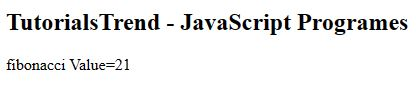﻿ JavaScript Fibonacci Program - TutorialsTrend
This Angular tutorial helps you get started with Angular quickly and effectively through many practical examples.
`

# JavaScript Fibonacci Program

#### Fibonacci Series Program

This program generates the Fibonacci series up to a given number. The fibonacci series numbers are given as: 0, 1, 1, 2, 3, 5, 8, 13, 21. In a Fibonacci series, every term is the sum of the preceding two terms, starting from 0 and 1 as the first and second terms.

Program

``````<!DOCTYPE html>
<html>
<title></title>
<body>
<h2>TutorialsTrend - JavaScript Programes</h2>
<script>

function fibonacci(num) {
let a = 0, b = 1, temp;
while (num >= 0) {
temp = a; a = a + b; b = temp; num--;
} return b;
}
document.write("fibonacci Value=" +fibonacci(8)); // 21
</script>
</body>
</html>``````

OutputPrev Next